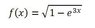# Taylor polynom of f(x)=1/(√1-e3x)

• Susenkovykral
In summary, the function you wrote does not have a Maclauren expansion and you copied it incorrectly.f

#### Susenkovykral

Member warned about posting homework/exam problems with no effort shown
Hello,

I can't find solution for Maclaurin (Taylor a=0) polynom of function: f(x)=1/(√1-e3x).

Thank you so much for help

Andrea

I think something went wrong with formatting. I guess you didn't mean$$f(x) = \frac 1 {\sqrt 1 - e3x}$$
Is this homework?

I'm sorry. I made wrong formattion. The function is in the picture http://file:///C:/Users/nemkv/Desktop/function.JPG [Broken]

It is from the exams.

#### Attachments

•function.JPG
2.4 KB · Views: 409
Last edited by a moderator:
I moved the thread to the homework section.
$$f(x)=\sqrt{1-e^{3x}}$$
Where did you run into problems?

Edit: Fixed sign. With minus it is odd.

Last edited:
The function you wrote, its wrong, it is with minus, not with plus.
My problem starts whan I make first (second, third, ..)derivation of this function and then I change x=a, and decide a=0.
When I decided a=0 the denominator equals 0. And I can't divide by zero. So I have no results.

The function you wrote, its wrong, it is with minus, not with plus.
My problem starts whan I make first (second, third, ..)derivation of this function and then I change x=a, and decide a=0.
When I decided a=0 the denominator equals 0. And I can't divide by zero. So I have no results.

The function ##f(x) = \sqrt{1-e^{3x}}## does not have a series expansion in powers of ##x-a## when ##a = 0## (that is, a Maclauren expansion). The function ##f(x)## is real when ##x < 0## but is pure imaginary when ##x > 0##, and there is no way any function like that can have a Maclauren expansion.

This is either a "trick" question or else you copied it incorrectly.

Last edited by a moderator:
Thank you so much. I have checked the function and I have copied it correctly. So It really looks like a trick.